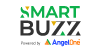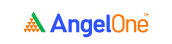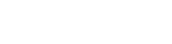# How to Understand Option Greeks

4.1In the last chapter, we saw the practical side of options trading. And now, it’s time to strengthen your theoretical awareness a little more. That’s because in this chapter, we’re going to discuss the options Greeks segment of options theory. Wondering what this is all about? Come, let’s see.

## Options Greeks explained: What are the Greeks of options theory?

Also referred to as options Greeks, these are basically different metrics that help traders understand how the price of an options contract reacts to various influencing factors. There are three main options Greeks, as listed here.

• Delta
• Gamma
• Theta

Now, they may appear to be complex concepts, but when you break down each idea, you’ll see it’s pretty simple. Let’s get right into the first Greek metric – the Delta.

### Delta of an options contract

You know that an options contract is a derivative. That means it derives its value from the underlying asset. So, if the price of the underlying asset changes, the price of the options contract (or its premium) will also change, right? This change in the premium of an options contract is what the delta measures.

### The definition of options delta

Technically, the delta of an options contract is defined as the rate of change in the price of the option with regard to changes in the price of the underlying asset. It can also be explained as the expected change in the price of an option relative to 1-rupee movements in the price of the underlying asset. It represents the directional risk of an option.

### Understanding options delta

What do we mean by directional risk? Well, it basically means that the delta shows how much the price of an option will change, when the price of the underlying asset moves in a certain direction (up or down).

• For a call option, the option price will increase as the underlying asset’s price increases (and vice versa).
• For a put option, the option price will decrease as the underlying asset’s price increases (and vice versa).

In other words, the call option’s price moves in the direction of the underlying asset’s price, while the put option’s price moves in the direction opposite to the underlying asset’s price.

Here’s an example to make things clearer. Consider call and put options with the shares of Godrej Consumer Products as the underlying asset.

 A B C D E Type of option Option price (in rupees) Option delta Option price if the share price increases by Re. 1 (in rupees) (B+C) Option price if the share price decreases by Re. 1 (in rupees) (B-C) Call 5.00 +0.75 5.75 4.25 Call 2.50 +0.30 2.80 2.20 Put 3.00 -0.25 2.75 3.25 Put 4.00 -0.60 3.40 4.60

### Directional movement of delta

• The delta of a call option always lies between 0 to 1 (same direction movement).
• And the delta of a call option always lies between 0 to -1 (opposite direction movement).

Looking at the table above, you’re perhaps wondering about why the value of options delta is limited to 1 on either side? That’s easy. You see, the price of the option is derived from the price of the asset, isn’t it? So, naturally, the derivative’s price cannot change more than the price of the asset, right?

For instance, take the call option from the above example and say that the share price of Godrej Consumer Products increases by Re. 1. Now, the call option, which derives its value from the Godrej Consumer Products share, cannot increase by more than Re. 1, right? Similarly, take the put option from the above example and say that the share price of Godrej Consumer Products increases by Re. 1. Now, the put option, which derives its value from the Godrej Consumer Products share, cannot decrease by more than Re. 1.

In other words, the derivative cannot gain or lose more than the underlying asset. That’s why the options delta is limited between 0 and 1 for calls and between o and -1 for puts. Here’s a visual depiction of this information.### Using delta to assess directional risk

Let’s take two call options, with details as follows.

 A B C D Option price (in rupees) Delta Option price if the share price increases by Re. 1 (in rupees) (A+B) Option price if the share price decreases by Re. 1 (in rupees) (A-B) 10 +0.90 10.90 9.10 2 +0.10 2.10 1.90

We can see that for the same level of share price movement, the call option with the higher delta fluctuates more in price while the call with the lower delta fluctuates less. So, options with higher deltas have more directional risk, since their values fluctuate more for each 1-rupee change in the underlying share’s price.

## Gamma of an options contract

The delta of an options contract keeps fluctuating. And it does not fluctuate at a linear rate. So, how do you assess this change in delta? That’s where gamma helps us.

### The definition of options gamma

The gamma of an options contract is defined as the rate of change in the delta of the option with regard to changes in the price of the underlying asset. In other words, it’s the expected change in the delta of an option relative to 1-rupee movements in the price of the underlying asset. It represents the change in an option’s directional risk.

### Understanding gamma

To understand gamma, we need to first see how the delta of an option changes when the price of the underlying asset moves. This is the general trend for the change in delta.

• For a call option, the delta will increase when the price of the asset increases (and vice versa).
• For a put option, the delta will decrease when the price of the asset increases (and vice versa).

In other words, the call option’s delta moves in the direction of the underlying asset’s price, while the put option’s delta moves in the direction opposite to the underlying asset’s price.

Here’s an example to make things clearer. Again, let’s consider call and put options with the shares of Godrej Consumer Products as the underlying asset.

 A B C D E Type of option Original delta Gamma New delta if the share price increases by Re. 1 (B+C) New delta if the share price decreases by Re. 1 (B-C) Call +0.50 +0.05 +0.55 +0.45 Call +0.10 +0.02 +0.12 +0.08 Put -0.25 +0.04 -0.21 -0.29 Put -0.40 +0.10 -0.30 -0.50

So, in the table above, you can see that when the share price increases, the delta of a call also goes up, while the delta of a put falls. Here, in the case of the first put option, the delta changes from -0.25 to -0.21. Mathematically speaking, you may assume that this means the delta increases (since negative 25 is less than negative 21 in math).

However, you need to keep in mind that the negative sign here is merely qualitative, since it indicates the direction of the movement only. So, -0.25 is higher than -0.21 here, because it denotes a higher rate of change.

### Visualizing gamma for call and put options

We saw how the delta changes for call and put options as the share price changes. But what does this movement denote? Let’s understand the changes in delta with a visual description.Note in the image above how call options become more sensitive when the stock price increases, while put options are less sensitive. This means that when the price of the stock increases by Re. 1, the delta of a call option will increase by more than by what the delta of a put option will decrease.

Conversely, when the stock price decreases, put options become more sensitive to stock price changes, while call options are less sensitive. This means that when the price of the stock decreases by Re. 1, the delta of a put option will increase by more than by what the delta of a call option will decrease.

## Theta of an options contract

In chapter 5 of this module, we saw what the intrinsic value of an option is, remember? Now, the premium that you pay for an options contract consists of two components - the extrinsic value and the intrinsic value.

 Premium of an option = extrinsic value + intrinsic value

The extrinsic value is composed of the time value of an option and the implied volatility. Now, as the expiry date of the contract approaches, the time value of an option decreases. So, the extrinsic value falls. And as a result, the premium (or option price) also falls. This is known as time decay, and the option theta helps gauge this metric.

### Why does the value of an option decrease over time?

Say the spot price of NIFTY is 9000. You buy a NIFTY call with a strike price of 9200. So, to be profitable to you, the NIFTY spot must move up to 9200, right? Let’s look at various timelines for this.

• Say you have 30 days for that call to expire. So, what’s the probability that the NIFTY spot will move up by 200 points over 30 days? Quite high.
• Now, say there are 10 days to expiry. What are the chances that the NIFTY spot will touch 9200 in that time? A little high, maybe, but not very much.
• If the expiry is closer, say within 5 days, then how likely is it that the NIFTY spot will go up to 9200? Not very likely.
• And what about the chances 1 day before expiry? They’re very low.

So, it’s evident that as the expiry draws closer, the chances of an option expiring in the money decrease. This is why its value also goes down.

### The definition of options theta

Theta is defined as the decay in the price of an option each day as the expiry draws closer. It helps measure the expected fall in the option premium with every passing day. Let’s take a look at an example to understand this concept better.

 A B C D Initial price of the option (in rupees) Theta Expected option price after 1 day (A-B) Expected option price after 10 days [A-(B x 10)] 10.00 -0.25 9.75 7.50 2.50 -0.05 2.45 2.00 1.00 -0.01 0.99 0.90

See how the price of the option reduces as the days pass? This is, of course, hypothetically assuming there’s no change in the spot price and therefore, in the intrinsic value. The theta is always negative because it represents a fall in the option’s price.

### Theta exposure of options

The options theta depends on the extrinsic value of the option. The higher the extrinsic value, the higher theta is. Let’s look at a theoretical example to see this in action.

Say you purchase 3 call options for shares with a market price of Rs. 200 each. Each call option has 45 days to expiry. Here are the other details

 A B C D E Strike price of the call option  (in rupees) Option price (premium)  (in rupees) Intrinsic value in rupees (market price - strike price) (zero, if intrinsic value is negative) Extrinsic value (in rupees) (B-C) Theta 1 150 (ITM) 60 50 10 -0.03 2 200 (ATM) 20 0 20 -0.07 3 240 (OTM) 5 0 5 -0.01

As you can see, the theta is higher for options where the extrinsic value is higher.

## Wrapping up

These are just some of the many Greeks used in relation to options. And with that, we have the fundamentals of options Greeks explained. But broadly speaking, they’re among the most essential concepts to know about, particularly if you’re getting started with options trading. Now that we’ve learned a lot about options, it’s time to head to the futures market. Visit the next chapter for more details about this.

## A quick recap

• Options Greeks are basically different metrics that help traders understand how the price of an options contract reacts to various influencing factors.
• There are three main Greeks: Delta, Gamma and Theta.
• The delta of an options contract is defined as the rate of change in the price of the option with regard to changes in the price of the underlying asset.
• It can also be explained as the expected change in the price of an option relative to 1-rupee movements in the price of the underlying asset.
• It represents the directional risk of an option.
• For a call option, the option price will increase as the underlying asset’s price increases (and vice versa).
• For a put option, the option price will decrease as the underlying asset’s price increases (and vice versa).
• The delta of a call option always lies between 0 to 1. And the delta of a call option always lies between 0 to -1.
• The gamma of an options contract is defined as the rate of change in the delta of the option with regard to changes in the price of the underlying asset.
• It’s the expected change in the delta of an option relative to 1-rupee movements in the price of the underlying asset. It represents the change in an option’s directional risk.
• For a call option, the delta will increase when the price of the asset increases (and vice versa).
• For a put option, the delta will decrease when the price of the asset increases (and vice versa).
• The premium that you pay for an options contract consists of two components - the extrinsic value and the intrinsic value.
• The extrinsic value is composed of the time value of an option and the implied volatility.
• As the expiry date of the contract approaches, the time value of an option decreases. So, the extrinsic value falls. And as a result, the premium (or option price) also falls. This is known as time decay, and the option theta helps gauge this metric.Take the quiz for this chapter & mark it complete.

How would you rate this chapter?

Get Information Mindfulness!

Catch-up With Market

News in 60 Seconds.The perfect starter to begin and stay tuned with your learning journey anytime and anywhere.